# 《C++ Primer》习题参考答案：第6章 - C++模块设计——函数

## 专栏C++学习笔记

《C++ Primer》学习笔记/习题答案 总目录

——————————————————————————————————————————————————————

# 第6章 - C++模块设计——函数

## 练习6.2

(a) int f() {
string s;
// ...
return s;
}
(b) f2(int i) { /* ... */ }
(c) int calc(int v1, int v1)  /* ... */ }
(d) double square (double x)  return x * x;


(a)是错误的，因为函数体返回的结果类型是 string，而函数的返回值类型是 int，二者不一致且不能自动转换。

string f() {
string s;
// ...
return s;
}


(b)是错误的，因为函数缺少返回值类型。如果该函数确实不需要返回任何值，则程序应该修改为：

void f2(int i) { /* ... */ }


int calc(int v1, int v2) { /* ... */ return ; }


(d)是错误的，因为函数体必须放在一对花括号内。

double square (double x) { return x * x; }


## 练习6.3

int fact(int i)
{
if (i < 0)
return -1;
int sum = 0;
sum = i > 1 ? i * fact(i - 1) : 1;

return sum;
}


## 练习6.4

#include <iostream>
using namespace std;
int fact(int i)
{
if (i < 0)
return -1;
int sum = 0;
sum = i > 1 ? i * fact(i - 1) : 1;

return sum;
}
int main()
{
int val, sum = 0;
cin >> val;
cout << val << " 的阶乘是 " << fact(val) << endl;

system("pause");
return 0;
}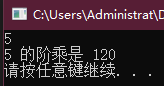## 练习6.5

#include <iostream>
#include <cmath>
using namespace std;
double myABS(double val)
{
if (val < 0)
return val * -1;
else
return val;
}
double sysABS(double val)
{
return abs(val);
}
int main()
{
double num;
cout << "请输入一个数：";
cin >> num;
cout << num << " 的 myABS 绝对值是 " << myABS(num) << endl;
cout << num << " 的 sysABS 绝对值是 " << sysABS(num) << endl;

system("pause");
return 0;
}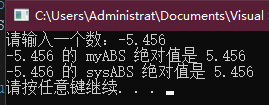myABS 函数使用 if-else 分支语句判断实参是正数还是负数，从而计算实参的绝对值。sysABS 函数直接调用 cmath 头文件的 abs 函数实现同样的功能。

## 练习6.6

• 形参是一种自动对象，函数开始时为形参申请内存空间，我们调用函数时提供的实参初始化形参对应的自动对象。
• 普通变量对应的自动对象也容易理解，我们在定义该变量的语句处创建自动对象，如果定义语句提供了初始值，则用该值初始化；否则，执行默认初始化。当该变量所在的块结束后，变量失效。
• 局部静态变量比较特殊，它的生命周期贯穿函数调用及之后的时间。局部静态变量对应的对象称为局部静态对象，它的生命周期从定义语句处开始，直到程序结束才终止。

#include <iostream>
using namespace std;
double myAdd(double val1, double val2){		// val1和val2是形参
double result = val1 + val2;			// result是普通局部变量
static unsigned iCnt = 0;				// iCnt是静态局部变量
++iCnt;
cout << "该函数已经累计执行了" << iCnt << "次" << endl;
return result;
}
int main(){
double num1, num2;
cout << "请输入两个数：";
while (cin >> num1 >> num2)
{
cout << num1 << "与" << num2 << "的求和结果是："
}

system("pause");
return 0;
}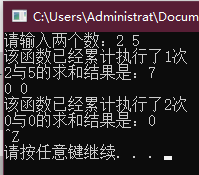## 练习6.7

#include <iostream>
using namespace std;
unsigned myCnt(){
static unsigned iCnt = -1;
++iCnt;
return iCnt;
}
int main(){
cout << "请输入任意字符后按回车键继续" << endl;
char ch;
while (cin >> ch)
{
cout << "函数myCnt（）执行的次数是：" << myCnt() << endl;
}

system("pause");
return 0;
}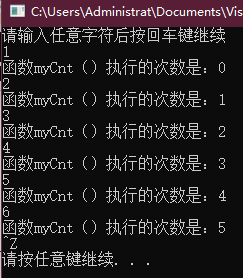## 练习6.8

#ifndef CHAPTER6_H_INCLUDED
#define CHAPTER6_H_INCLUDED

int fact(int);
double myABS(double);
double sysABS(double);

#endif // CHAPTER6_H_INCLUDED


## 练习6.9 : fact.cc | factMain.cc

fact.cc

#include "Chapter6.h"
using namespace std;
int fact(int val)
{
if (val < 0)
return -1;
int ret = 1;
for (int i = 1; i != val; ++i){
ret *= i;
}
return ret;
}


factMain.cc

#include <iostream>
#include "Chapter6.h"
using namespace std;
int main()
{
int num;
cout << "请输入一个数：";
cin >> num;
cout << num << " 的阶乘是：" << fact(num) << endl;

system("pause");
return 0;
}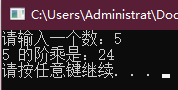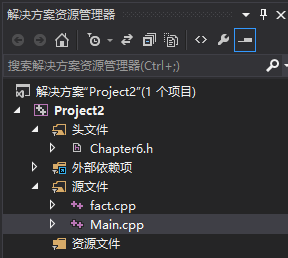## 练习6.10

#include <iostream>
using namespace std;
// 在函数体内部通过解引用操作改变指针所指的内容
void mySwap(int *p, int *q){
int tmp = *p;
*p = *q;
*q = tmp;
}
int main(){
int a = 5, b = 10;
int *r = &a, *s = &b;
cout << "交换前：a = " << a << "，b = " << b << endl;
// 指针形参
mySwap(r, s);
// 引用形参（建议）
// mySwap(&a, &b);
cout << "交换后：a = " << a << "，b = " << b << endl;

system("pause");
return 0;
}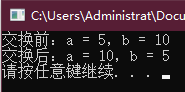// 在函数体内部交换了两个形参指针本身的值，未能影响实参
void mySwap(int *p, int *q){
int *tmp = p;
p = q;
q = tmp;
}


## 练习6.11

#include <iostream>
using namespace std;
void reset(int &i){
i = 0;
}
int main(){
int num = 5;
cout << "重置前：num = " << num << endl;
reset(num);
cout << "重置前：num = " << num << endl;

system("pause");
return 0;
}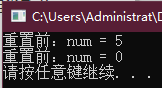## 练习6.12

#include <iostream>
using namespace std;
void mySwap(int &p, int &q){
int tmp = p;
p = q;
q = tmp;
}
int main(){
int a = 5, b = 10;
int *r = &a, *s = &b;
cout << "交换前：a = " << a << "，b = " << b << endl;
mySwap(a, b);
cout << "交换后：a = " << a << "，b = " << b << endl;

system("pause");
return 0;
}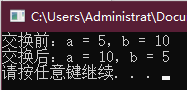## 练习6.13

• void f(T) 的形参采用的是传值方式，也就是说，实参的值被拷贝给形参，形参和实参是两个相互独立的变量，在函数 f 内部对形参所做的任何改动都不会影响实参的值。

• void f(&T) 的形参采用的是传引用方式，此时形参是对应的实参的别名，形参绑定到初始化它的对象。如果改变了形参的值，也就是改变了对应实参的值。

#include <iostream>
using namespace std;
void a(int);
void b(int&);
int main(){
int s = 0, t = 10;
a(s);
cout << s << endl;
b(t);
cout << t << endl;

system("pause");
return 0;
}
void a(int i){
++i;
cout << i << endl;
}
void b(int& j){
++j;
cout << j << endl;
}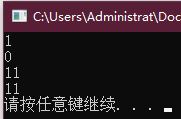## 练习6.14

• 一是可以直接操作引用形参所引的值；
• 二是使用引用形参可以避免拷贝大的类类型对象或容器类型对象；
• 三是使用引用形参可以帮助从函数中返回多个值。

• 第一，当函数的目的是交换两个参数的内容时，应该使用引用类型的形参；
• 第二，当参数是 string 对象时，为了避免拷贝很长的字符串，应该使用引用类型。

## 练习6.15find_char 函数的三个参数的类型设定与该函数的处理逻辑密切相关，原因分别如下：

• 对于待查找的字符串 s 来说，为了避免拷贝长字符串，使用引用类型；同时我们只执行查找操作，无须改变字符串的内容，所以将其声明为常量引用。
• 对于待查找的字符 c 来说，它的类型是 char ，只占1字节，拷贝的代价很低，而且我们无须操作实参在内存中实际存储的内容，只把它的值拷贝给形参即可，所以不需要使用引用类型。
• 对于字符出现的次数 occurs 来说，因为需要把函数内对实参值的更改反应在函数外部，所以必须将其定义成引用类型，但是不能把它定义成常量引用，否则就不能改变所引的内容了。

## 练习6.16

bool is_empty(string& s) { return s.empty(); }


• 一是容易给使用者一种误导，即程序允许修改 s 的内容；
• 二是限制了该函数所能接收的实参类型，我们无法把 const 对象、字面值常量或者需要进行类型转换的对象传递给普通的引用形参。
bool is_empty(const string& s) { return s.empty(); }


## 练习6.17

• 第一个函数的任务是判断 string 对象中是否有大写字母，无需修改参数的内容，因此将其设为常量引用类型。
• 第二个函数的任务是转换 string 对象中的字母为小写字母，需要修改参数的内容，所以应该将其设定为非常量引用类型。

#include <iostream>
#include <string>
using namespace std;
bool HasUpper(const string& str){
for (auto c : str)
if (isupper(c))
return true;
return false;
}
void ChangeToLower(string& str){
for (auto &c : str)
c = tolower(c);
}
int main(){
cout << "请输入一个字符串：" << endl;
string str;
cin >> str;
if (HasUpper(str)){
ChangeToLower(str);
cout << "转换后的字符串是：" << str << endl;
}
else{
cout << "该字符串不含大写字母，无需转换!" << endl;
}

system("pause");
return 0;
}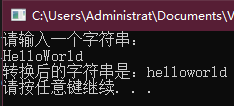## 练习6.18

(a) 名为 compare 的函数，返回布尔值，两个参数都是 matrix 类的引用。
(b) 名为 change_val 的函数，返回 vector 的迭代器，有两个参数：一个是 int，另一个是 vector 的迭代器。

(a)的函数声明是：

bool compare(const matrix&, matrix&);


(b)的函数声明是：

vector<int>::iterator change_val(int, vector<int>::iterator);


## 练习6.19

double calc(double);
int count(const string &, char);
int sum(vector<int>::iterator, vector<int>::iterator, int);
vector<int> vec(10);
(a) calc(23.4, 55.1);
(b) count("abcda",'a');
(c) calc(66);
(d) sum(vec.begin(), vec.end(), 3.8);


(a)是非法的，函数的声明只包含一个参数，而函数的调用提供了两个参数，因此无法编译通过。

(b)是合法的，字面值常量可以作为常量引用形参的值，字符 ‘a’ 作为 char 类型形参的值也是可以的。

©是合法的，66 虽然是 int 类型，但是在调用函数时自动转换为 double 类型。

(d)是合法的，vec.begin()vec.end() 的类型都是形参所需的 vector<int>::iterator，第三个实参 3.8 可以自动转换为形参所需的 int 类型。

## 练习6.20

• 一是容易给使用者一种误导，即程序允许修改实参的内容；
• 二是限制了该函数所能接受的实参类型，无法把 const 对象、字面值常量或者需要类型转换的对象传递给普通的引用形参。

## 练习6.21

#include <iostream>
#include <string>
#include <ctime>
using namespace std;
int myCompare(const int val, const int *p){
return (val > *p) ? val : *p;
}
int main(){
srand((unsigned)time(NULL));
int a;
for (auto &i : a)
i = rand() % 100;
cout << "请输入一个数：";
int j;
cin >> j;
cout << "您输入的数与数组首元素中较大的是：" << myCompare(j, a) << endl;
cout << "数组的全部元素是：" << endl;
for (auto i : a)
cout << i << " ";
cout << endl;

system("pause");
return 0;
}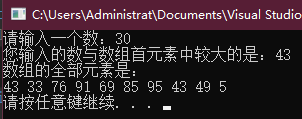## 练习6.22

• 第一个函数以值传递的方式使用指针，所有改变都局限于函数内部，当函数执行完毕后既不会改变指针本身的值，也不会改变指针所指的内容。
• 第二个函数同样以值传递的方式使用指针，但是在函数内部通过解引用的方式直接访问内存并修改了指针的方式。
• 第三个函数的参数形式是 int*&，其含义是，该参数是一个引用，引用的对象是内存中的一个 int 指针，使用这种方式可以把指针当成对象，交换指针本身的值。需要注意的是，最后一个函数既然交换了指针，当然解引用该指针所得的结果也会相应发生改变。
#include <iostream>
using namespace std;
// 该函数既不交换指针，也不交换指针所指的内容
void SwapPointer1(int *p, int *q){
int *temp = p;
p = q;
q = temp;
}
// 该函数交换指针所指的内容
void SwapPointer2(int *p, int *q){
int temp = *p;
*p = *q;
*q = temp;
}
// 该函数交换指针本身的值，即交换指针所指的内存地址
void SwapPointer3(int &p, int &q){
int tmp = p;
p = q;
q = tmp;
}
int main(){
int a = 5, b = 10;
int *p = &a, *q = &b;
cout << "交换前：" << endl;
cout << "p的值是：" << p << "，q的值是：" << q << endl;
cout << "p所指的值是：" << *p << "，q所指的值是：" << *q << endl;
SwapPointer1(p, q);
cout << "交换后：" << endl;
cout << "p的值是：" << p << "，q的值是：" << q << endl;
cout << "p所指的值是：" << *p << "，q所指的值是：" << *q << endl;

cout << endl;
cout << "*********************************************************" << endl;
cout << endl;

int c = 5, d = 10;
int *r = &c, *s = &d;
cout << "交换前：" << endl;
cout << "p的值是：" << r << "，q的值是：" << s << endl;
cout << "p所指的值是：" << *r << "，q所指的值是：" << *s << endl;
SwapPointer2(r, s);
cout << "交换后：" << endl;
cout << "p的值是：" << r << "，q的值是：" << s << endl;
cout << "p所指的值是：" << *r << "，q所指的值是：" << *s << endl;

cout << endl;
cout << "*********************************************************" << endl;
cout << endl;

int e = 5, f = 10;
int *x = &e, *y = &f;
cout << "交换前：" << endl;
cout << "p的值是：" << x << "，q的值是：" << y << endl;
cout << "p所指的值是：" << *x << "，q所指的值是：" << *y << endl;
//SwapPointer3(e, f);
SwapPointer3(*x, *y);
cout << "交换后：" << endl;
cout << "p的值是：" << x << "，q的值是：" << y << endl;
cout << "p所指的值是：" << *x << "，q所指的值是：" << *y << endl;

system("pause");
return 0;
}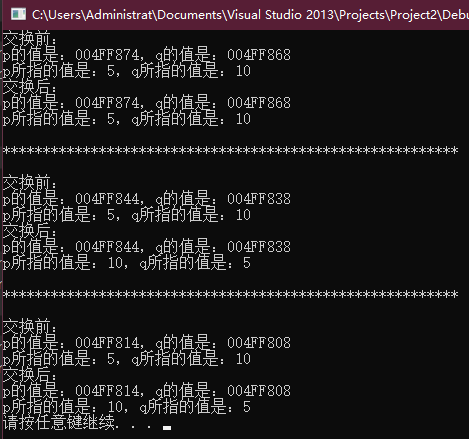## 练习6.23

int i = 0, j = { 0, 1 };


• 第一个版本不控制指针的边界，
• 第二个版本由调用者指定数组的维度，
• 第三个版本使用C++11新规定的 beginend 函数限定数组边界。

#include <iostream>
using namespace std;
// 参数是常量整型指针
void print1(const int *p){
cout << *p << endl;
}
// 参数有两个，分别是常量整型指针和数组的容量
void print2(const int *p, const int sz){
int i = 0;
while (i != sz) {
cout << *p++ << endl;
++i;
}
}
// 参数有两个，分别是数组的首尾边界
void print3 (const int *b, const int *e){
for (auto q = b; q != e; ++q){
cout << *q << endl;
}
}
int main(){
int i = 0, j = { 0, 1 };
print1(&i);
print1(j);

cout << endl;
cout << "*********************************************************" << endl;
cout << endl;

print2(&i, 1);
//计算得到数组j的容量
print2(j, sizeof(j) / sizeof(*j));

cout << endl;
cout << "*********************************************************" << endl;
cout << endl;

auto b = begin(j);
auto e = end(j);
print3(b, e);

system("pause");
return 0;
}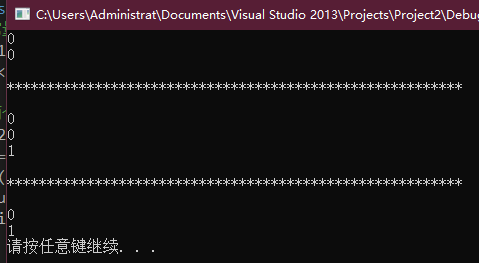## 练习6.24

void print(const int ia)
{
for (size_t i = 0; i != 10; ++i)
cout << ia[i] << endl;
}


• 一是声明为指针，
• 二是声明为不限维度的数组，
• 三是声明为维度确定的数组。

void print(const int ia[], const int sz)
{
for (size_t i = 0; i != sz; ++i)
cout << ia[i] << endl;
}


## 练习6.25

#include <iostream>
#include <string>
using namespace std;
int main(int argc, char **argv){
string str;
for (int i = 0; i != argc; ++i)
str += argv[i];
cout << str << endl;

system("pause");
return 0;
}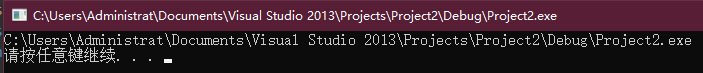• int argc 用来表示命令行参数的个数；
• char *argv[] 用来取得所输入的参数；

## 练习6.26

#include <iostream>
using namespace std;
int main(int argc, char **argv){
for (int i = 0; i != argc; ++i){
cout << "argc[" << i << "]：" << argv[i] << endl;
}

system("pause");
return 0;
}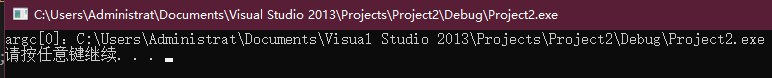## 练习6.27

#include <iostream>
#include <initializer_list>
using namespace std;
int iCount(initializer_list<int> il){
int count = 0;
// 遍历il的每一个元素
for (auto val : il)
count += val;
return count;
}
int main(){
// 使用列表初始化的方式构建initializer_list<int>对象
// 然后把它作为实参传递给函数iCount
cout << "1,6,9的和是：" << iCount({ 1, 6, 9 }) << endl;
cout << "4,5,9,18的和是：" << iCount({ 4, 5, 9, 18 }) << endl;
cout << "10,10,10,10,10,10,10,10,10的和是"
<< iCount({ 10, 10, 10, 10, 10, 10, 10, 10, 10 }) << endl;

system("pause");
return 0;
}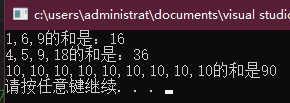## 练习6.28

error_msg 函数的第二个版本中包含 ErrCode 类型的参数，其中循环内的 elem 是什么类型？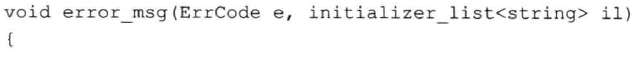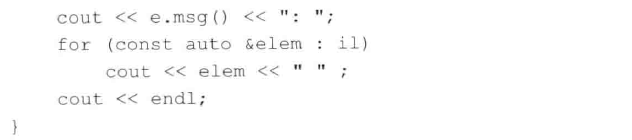initializer_list<string> 的所有元素类型都是 string，因此 const auto &elem : il 推断得到的 elem 的类型是 const strings

## 练习6.30

#include <iostream>
using namespace std;
bool str_subrange(const string &str1, const string &str2){
if (str1.size() == str2.size())
return str1 == str2;
auto size = (str1.size() < str2.size()) ? str1.size() : str2.size();
for (decltype(size)i = 0; i != size; ++i){
if (str1[i] != str2[i])
return;
}
}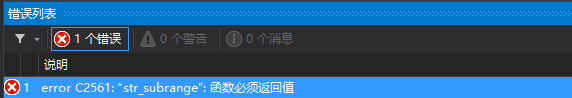## 练习6.31

• 如果返回的是值，则创建一个为命名的临时对象，并把要返回的值拷贝给这个临时对象；
• 如果返回的是引用，则该引用是它所引对象的别名，不会真正拷贝对象。
• 如果引用所引的是函数开始之前就已经存在的对象，则返回该引用是有效的；
• 如果引用所引的是函数的局部变量，则随着函数结束局部变量也失效了，此时返回的引用无效。

## 练习6.32

int &get(int *array, int index) { return array[index]; }
int main()
{
int ia;
for (int i = 0; i != 10; ++i)
get(ia, i) = i;
}


get 函数接受一个整型指针，该指针实际指向一个整型数组的首元素，另外还接受一个整数表示数组中某个元素的索引值。它的返回值类型是整型引用，引用的对象是 arry 数组的某个元素。当 get 函数执行完毕，调用者得到实参数组 arry 中索引为 index 的元素引用。

main 函数中，首先创建一个包含10个整数的数组，名字是 ia。请注意，由于 ia 定义在函数内部，所以 ia 不会执行默认初始化操作，如果此时我们直接输出 ia 每个元素的值，则这些值都是未定义的。

#include <iostream>
using namespace std;
int &get(int *array, int index) { return array[index]; }
int main()
{
int ia;
for (int i = 0; i != 10; ++i)
get(ia, i) = i;

for (int i = 0; i < 10; i++)
{
printf("%d ", ia[i]);
}
printf("\n");

system("pause");
//return 0;
}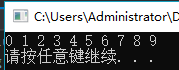## 练习6.33

#include <iostream>
#include <vector>
using namespace std;
void print(vector<int> vInt, unsigned index){
unsigned sz = vInt.size();
if (!vInt.empty() && index < sz){
cout << vInt[index] << endl;
print(vInt, index + 1);
}
}
int main()
{
vector<int> v{ 1, 3, 5, 7, 9, 11, 13, 15 };
print(v, 0);

system("pause");
return 0;
}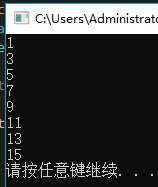## 练习6.34

if (val != 0)


## 练习6.35

int factorial(int val){
if (val < 1)
return factorial(val-1) * val;
return 1;
}


## 练习6.36

string (&func());


func() 表示调用 func 函数无须任何实参，(&func()) 表示函数的返回结果是一个引用，(&func()) 表示引用的对象是一个维度为10的数组，string (&func()) 表示数组的元素是 string 对象。

## 练习6.37

typedef string arr;
arr& func();


auto func()->string(&) ;


string str;
decltype(str)& func();


## 练习6.38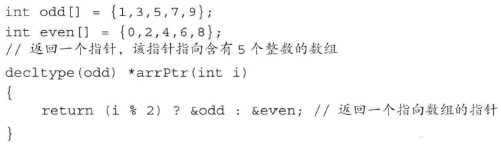int odd[] = {1, 3, 5, 7, 9};
int even[] = {0, 2, 4, 6, 8};
// 返回一个指针，该指针指向含有5个整数的数组
decltype(odd) &arrPtr(int i)
{
return (i % 2) ? odd : even;  // 返回数组的引用
}


## 练习6.39

(a) int calc(int, int);
int calc(const int, const int);
(b) int get();
double get();
(c) int *reset(int *);
double *reset(double *);


(a)的第二个声明是非法的。它的意图是声明另外一个函数，该函数只接受整型常量作为实参，但是因为 顶层 const 不影响传入函数的对象，所以一个拥有顶层 const 的形参无法与另一个没有顶层 const 的形参区分开来。

(b)的第二个声明是非法的。它的意图是通过函数的返回值区分两个同名的函数，但是这不可行，因为 C++规定重载函数必须在形参数量或形参类型上有所区别。如果两个同名函数的形参数量和类型都一样，那么即使返回类型不同也不行。

## 练习6.40

(a) int ff(int a, int b = 0, int c = 0);
(b) char *init(int ht = 24, int wd, char bckgrnd);


## 练习6.41

char *init(int ht, int wd = 80, char bckgrnd = ' ');
(a) init();
(b) init(24,10);
(c) init(14,'*');


(a)是非法的，该函数有两个默认实参，但是总计有三个形参，其中第一个形参并未设定默认实参，所以要想调用该函数，至少需要提供一个实参。

(b)是合法的，本次调用提供了两个实参，第一个实参对应第一个形参 ht，第二个实参对应第二个形参 wd，其中 wd 的默认实参没有用到，第三个形参 bckgrnd 使用它的默认实参。

©在语法上是合法的，但是与程序的原意不符。从语法上来说，第一个实参对应第一个形参 ht，第二个实参的类型虽然是 char，但是它可以自动转换为第二个形参 wd 所需的 int 类型，所以编译时可以通过，但这显然违背了程序的原意，正常情况下，字符 * 应该被用来构成 bckgrnd

## 练习6.42

make_plural 函数的第二个形参赋予默认实参’s’, 利用新版本的函数输出单词 successfailure 的单数和复数形式。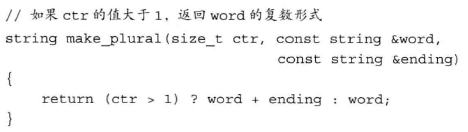#include<iostream>
#include<string>
using namespace std;
// 最后一个形参赋予了默认实参
string make_plural(size_t ctr, const string &word, const string &ending = "s")
{
return (ctr > 1) ? word + ending : word;
}
int main(){
cout << "success的单数形式是：" << make_plural(1, "success", "es") << endl;
cout << "success的复教形式是：" << make_plural(2, "success", "es") << endl;
// 一般情况下调用该函数只需要两个实参
cout << "failure的单数形式是：" << make_plural(1, "failure") << endl;
cout << "failure的单数形式是：" << make_plural(2, "failure") << endl;

system("pause");
return 0;
}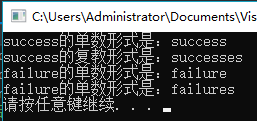## 练习6.43

(a) inline bool eq(const BigInt&, const BigInt&) {...}
(b) void putValues(int *arr, int size);


(a)应该放在头文件中。因为内联函数的定义对编译器而言必须是可见的，以便编译器能够在调用点内联展开该函数的代码，所以仅有函数的原型不够。

(b)是函数声明，应该放在头文件中。

## 练习6.44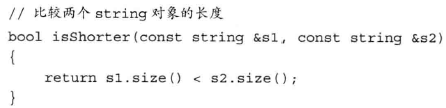inline bool isShorter(const string &s1, const string &s2)
{
return s1.size() < s2.size();
}


## 练习6.45

• 练习6.11中的 reset 函数改写后的形式是：
inline void reset(int &i)
{
i=0;
}

• 练习6.21中的 myCompare 函数改写后的形式是：
inline int mycompare(const int val, const int *p)
{
return (val > *p) ? val : *p;
}


## 练习6.46

constexpr 函数是指能用于常量表达式的函数，constexpr 函数的返回类型和所有形参的类型都得是字面值类型，而且函数体中必须有且只有一条 return 语句。

## 练习6.47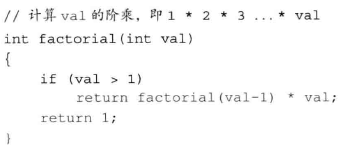#include<iostream>
#include<vector>
using namespace std;
// 递归函数输出vector<int>的内容
void print(vector<int> vInt, unsigned index)
{
unsigned sz = vInt.size();
// 设置在此处输出调试信息
#ifndef NDEBUG
cout << "vector对象的大小是：" << sz << endl;
#endif	// NDEBUG
if(!vInt.empty() && index < sz)
{
cout << vInt[index] << endl;
print(vInt, index + 1);
}
}
int main()
{
vector<int> v = { 1, 3, 5, 7, 9, 11, 13, 15 };
print(v, 0);

system("pause");
return 0;
}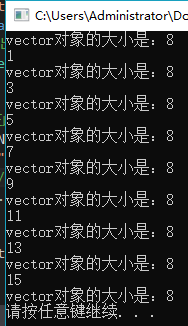## 练习6.48

string s;
while (cin >> s && s != sought) { } // 空函数体
assert(cin);


assert(s==sought);


## 练习6.49

• 一是与被调用的函数同名，
• 二是其声明在调用点可见。

• 一是其形参数量与本次调用提供的实参数量相等，
• 二是每个实参的类型与对应的形参类型相同或者能转换成形参的类型。

## 练习6.50

(a) f(2.56, 42)
(b) f(42)
(c) f(42, 0)
(d) f(2.56, 3.14)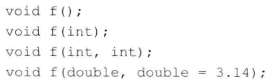• 可行函数是指形参数量与本次调用提供的实参数量相等且每个实参的类型都与对应的形参类型相同或者能转换成形参类型的函数。
• 最佳匹配是指该函数每个实参的匹配都不劣于其他可行函数需要的匹配且至少有一个实参的匹配优于其他可行函数提供的匹配。

• f(2.56, 42) 的可行函数是 void f(int, int)void f(double, double=3.14)。但是最佳匹配不存在，因为这两个可行函数各有所长。对于这次调用来说，如果只考虑第一个实参 2.56，我们发现，void f(double, double=3.14) 能够精确匹配，但是要想匹配第二个参数，int 类型的实参 42 必须转换成 double 类型。如果考虑第二个实参 42，我们发现，void f(int, int) 能够精确匹配，但是要想调用 void f(int, int) 就必须把第一个 double 类型的实参 2.56 转换成 int 类型。最终的结果是这两个可行函数各自在一个实参上实现了更好的匹配，但是把它们比较起来无从判断孰优孰劣，因此编译器将因为这个调用具有二义性而拒绝其请求。

• f(42) 的可行函数是 void f(int)void f(double, double=3.14)，其中最佳匹配是 void f(int)，因为参数无须做任何类型转换。

• f(42, 0) 的可行函数是 void f(int, int)void f(double, double=3.14)，其中最佳匹配是 void f(int, int)，因为参数无须做任何类型转换。

• f(2.56, 3.14) 的可行函数是 void f(int, int)void f(double, double=3.14)，其中最佳匹配是 void f(double, double=3.14)，因为参数无须做任何类型转换。

## 练习6.51

#include<iostream>
using namespace std;
void f(){
cout << "该函数无须参数" << endl;
}
void f(int){
cout << "该函数有一个整型参数" << endl;
}
void f(int, int){
cout << "该函数有两个整型参数" << endl;
}

void f(double a, double b = 3.14){
cout << "该函数有两个双精度浮点型参数" << endl;
}

int main(){
//f(2.56, 42);	// 报错
f(42);
f(42, 0);
f(2.56, 3.14);

system("pause");
return 0;
}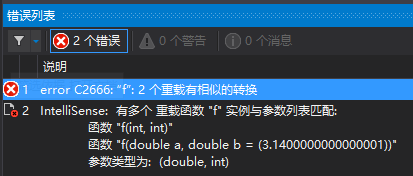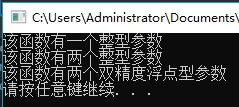## 练习6.52

void manip(int ,int);
double dobj;


(a) manip('a', 'z');
(b) manip(55.4, dobj);


(a)发生的参数类型转换是类型提升，字符型实参自动提升成整型。

(b)发生的参数类型转换是算术类型转换，双精度浮点型自动转换成整型。

## 练习6.53

(a) int calc(int&, int&);
int calc(const int&, const int&);
(b) int calc(char*, char*);
int calc(const char*, const char*);
(c) int calc(char*, char*);
int calc(char* const, char* const);


(a)是合法的，两个函数的区别是它们的引用类型的形参是否引用了常量，属于底层 const，可以把两个函数区分开来。

(b)是合法的，两个函数的区别是它们的指针类型的形参是否指向了常量，属于底层 const，可以把两个函数区分开来。

©是非法的，两个函数的区别是它们的指针类型的形参本身是否是常量，属于顶层 const，根据本节介绍的匹配规则可知，向实参添加顶层 const 或者从实参中删除顶层 const 属于精确匹配，无法区分两个函数。

## 练习6.54

int func(int, int);

vector<decltype(func)* > vF;


## 练习6.55

#include<iostream>
#include<vector>
using namespace std;
int func1(int a, int b){
return a + b;
}
int func2(int a, int b){
return a - b;
}
int func3(int a, int b){
return a * b;
}
int func4(int a, int b){
return a / b;
}
int main(){
decltype(func1) *p1 = func1, *p2 = func2, *p3 = func3, *p4 = func4;
vector<decltype(func1)*>vF = { p1, p2, p3, p4 };

system("pause");
return 0;
}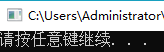## 练习6.56

#include<iostream>
#include<vector>
using namespace std;
int func1(int a, int b){
return a + b;
}
int func2(int a, int b){
return a - b;
}
int func3(int a, int b){
return a * b;
}
int func4(int a, int b){
return a / b;
}
void Compute(int a, int b, int(*p)(int, int)){
cout << p(a, b) << endl;
}
int main(){
int i = 5, j = 10;
decltype(func1) *p1 = func1, *p2 = func2, *p3 = func3, *p4 = func4;
vector<decltype(func1)*>vF = { p1, p2, p3, p4 };
// 遍历vector中的每个元素，依次调用四则运算函数
for (auto p : vF){
Compute(i, j, p);
}

system("pause");
return 0;
}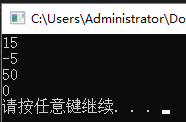11-2303-06290
08-311507
08-032585
09-253052
04-10245
03-08150
07-06133